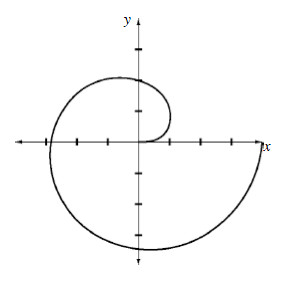### Home > CALC > Chapter 12 > Lesson 12.2.3 > Problem12-94

12-94.

Multiple Choice: Without a calculator, find the area of the region in the second and third quadrants bounded by the polar curve $r ( \theta ) = \sqrt { \frac { 8 \theta } { \pi } }$and the y-axis, as shown at right.

1. $4π$

1. $8π$

1. $\frac { 2 \pi } { 3 } ( 3 ^ { 3 / 2 } - 1 )$

1. $\frac { 13 \pi ^ { 3 } } { 24 }$

1. $\frac { 18 } { \pi }$